# What to Expect: Calculating Expected Values with Probability Distributions in Wolfram|Alpha

August 16, 2012 —Comments Off

In a previous post, I discussed Wolfram|Alpha’s facility with probability distributions, such as the distribution of probability among the possible totals that can be shown with a pair of fair dice: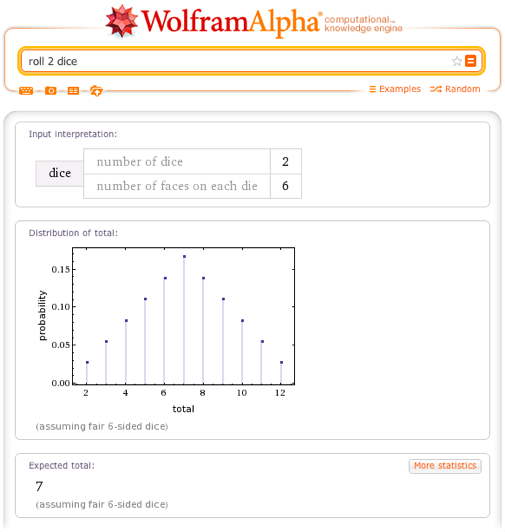If you rolled the dice many times, what would be your average roll? To compute this you would take the grand total of all your rolls and divide by the number of rolls, but we can get a good idea of what to expect using the distribution.

The heights of the bars above represent the approximate fraction of times you would observe each roll. So you would roll a total of 2 roughly 3% of the time, and the amount of the grand total contributed by the times you rolled a 2 would be roughly (2)(3%) times the number of rolls. We can apply the same reasoning to each of the other outcomes and can sum all the contributions to get the grand total. Dividing by the number of rolls to get the average gives us the sum (2)(3%) + (3)(6%) + (4)(8%) + …, which we call the expected value of the distribution. The value of this expression is 7, as can be seen in the “Expected total” pod above.

The effect of totaling the products of outcomes and probabilities is to create a weighted average of the outcomes with the probabilities providing the weights. This represents your approximate average over many rolls and is called the expected value of the probability distribution from which it came.

Expected value has a nice visual interpretation as the balance point of the distribution. If the horizontal axis above were a seesaw, the expected value would be the point where you’d have to put the fulcrum to get it to balance. For the distribution of the rolls of two dice, it is visually obvious that this point is 7.

Wolfram|Alpha can compute expected values of the many probability distributions that it knows. For example, the binomial distribution distributes probability among the possible counts of heads in n flips of a coin that is weighted so that the probability of a single flip landing heads is p: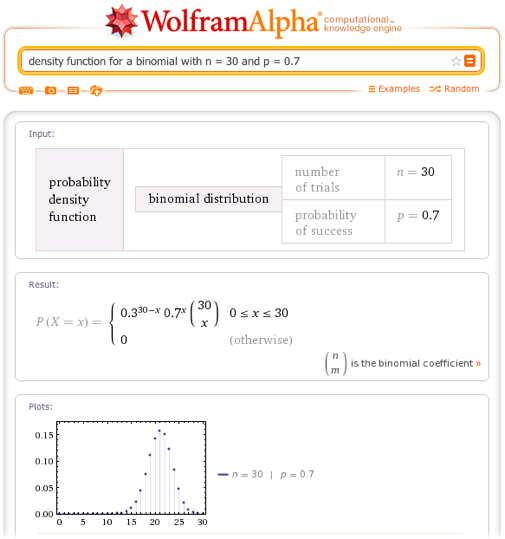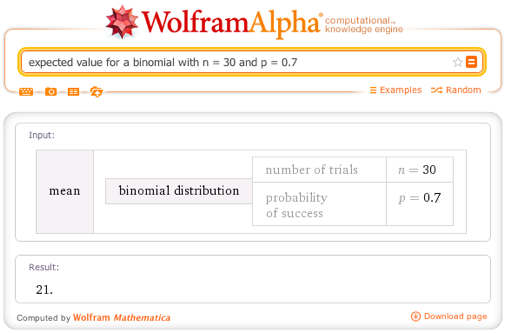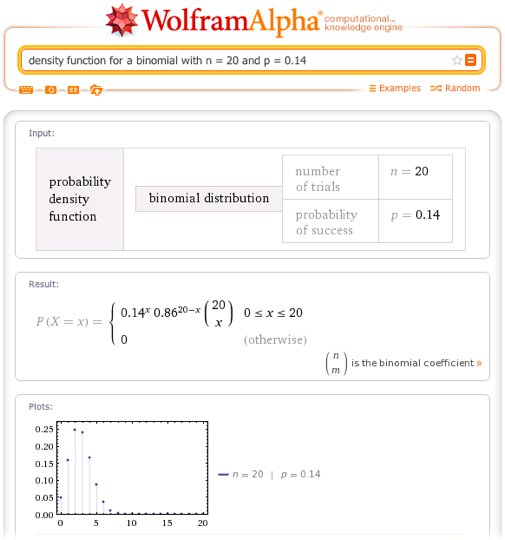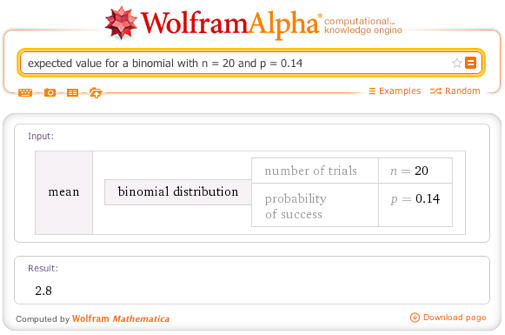For almost all named families of probability distributions, the expected value can be computed as a function of the parameters. We can get these formulas from Wolfram|Alpha, too: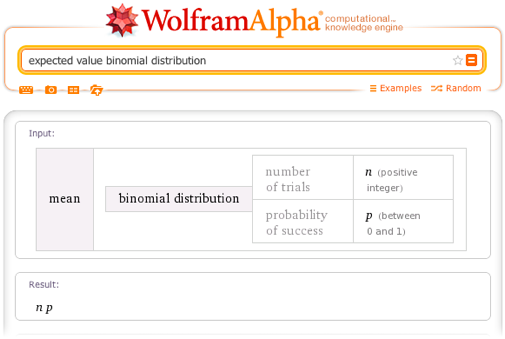This makes sense! If I flip n = 100 coins with p = 0.2 probability of heads on each flip, then I expect to get np = (100)(.2) = 20 heads.

For continuous distributions, the mathematical definition of the expected value is slightly more complicated, but with Wolfram|Alpha, this additional computational complexity is not an obstacle. What’s more, the interpretation of the expected value as the balance point of the distribution is still a good one. Looking at the standard normal distribution, we would guess that the expected value is 0…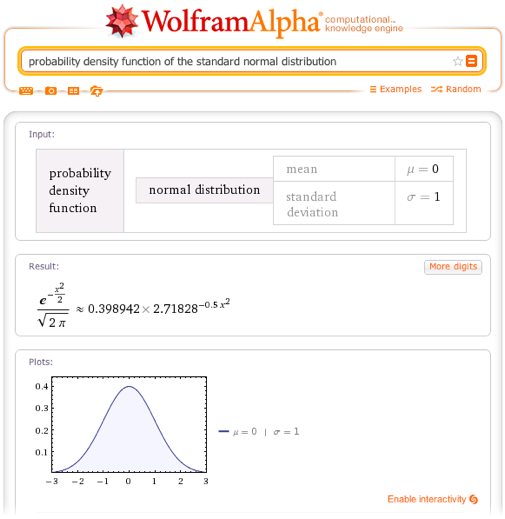…and indeed it is: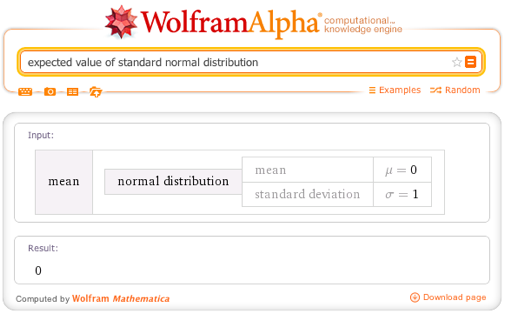We noted in the probability blog post that the parameter μ controls the center of a normal distribution.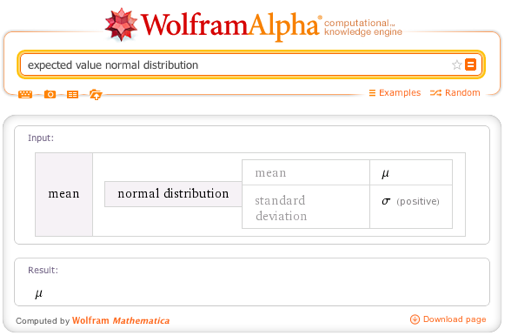Other distributions are not so symmetric: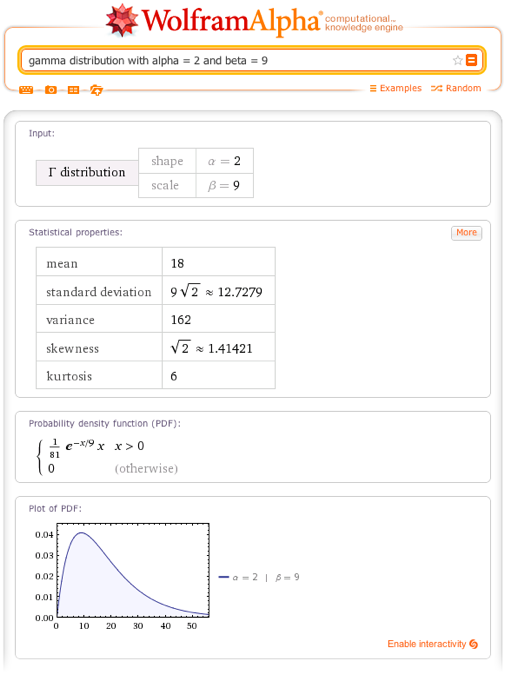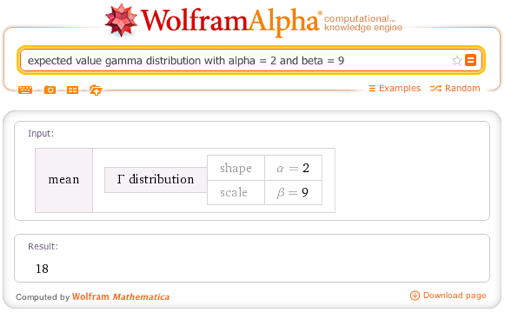It turns out that the expected value of a gamma distribution is the product of the distribution’s parameters: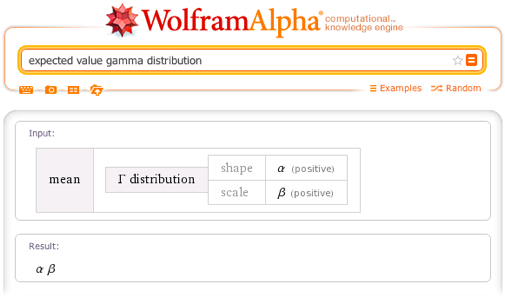In Wolfram|Alpha, you can specify some of a distribution’s parameters and see how the expected value is a function of the others: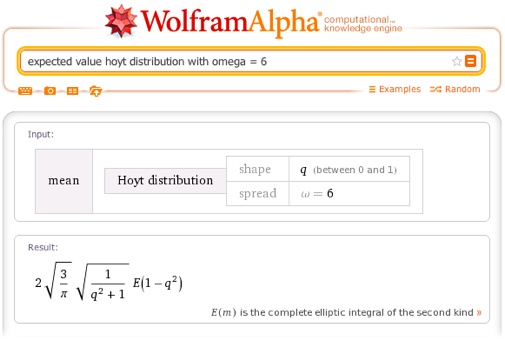Computing expected values often requires computing complicated sums or integrals. The burden of this requirement is eased somewhat by the existence of nice formulas for the expected values of many distributions. Wolfram|Alpha provides one place to do such computations easily, but where Wolfram|Alpha really offers a significant advance is in its ability to easily compute expected values of functions of distributions. Suppose you were to flip a fair coin ten times and square the number of heads you observe. What do you expect? Perhaps surprisingly, the answer is not 25: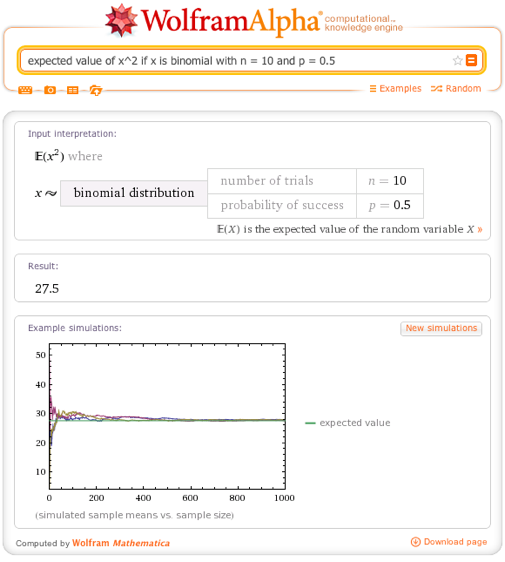The “Example simulations” pod above shows the results of a few runs of the experiment just described—flip the coin, count the heads, square the result, and average these numbers over many trials. These averages all move close to the computed expected value of 27.5 as the number of trials grows.

For other distributions, one can do similar thought experiments. Draw a value from the distribution (i.e., pick a “random” number, where the probability of an outcome is given by the probability distribution in question) and square it (or cube it, or multiply it by 7 and add 4, etc.)—what do we expect?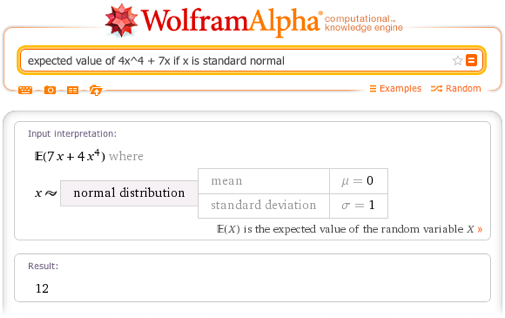These expected values can be quite complicated: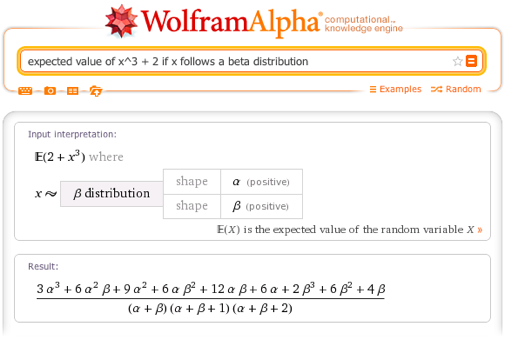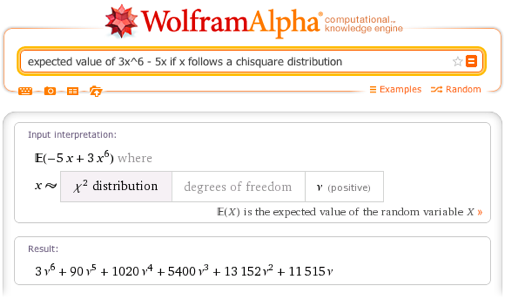Of course, we are eager to expand Wolfram|Alpha’s facility with expected values even further and look forward to getting feedback from you and bringing you more and better functionality in the future.

I’m new to wolfram alpha. Is there a way to determine on it, for example, the number of possible groups of size 3 (n=3) when you have a total of 6 members (N=6). The order of each group (or combination) does matter.

Thanks

Posted by David August 20, 2012 at 6:08 pm

Try “3-combinations of 6 objects”

Posted by Chris Boucher August 27, 2012 at 9:04 am

When I was studying probability in April and May those functionalities were quite broken, glad to see you’ve improved them!

Posted by Lazza August 26, 2012 at 7:23 am

what to type to calculate the probability distribution of a setup of different dice (4 [3x], 6 [2x], 8 [2x], 10, 12, 20 faces) given a rule to reroll (and add the previous roll) any dice at maximum. for example one 8- and 4-faced dice with result in 7 and 4,4,3 (the 4sided one maxed twice, had to be rerolled and therefor has the output of 11) ?

Posted by max August 26, 2012 at 9:05 am

You can investigate the distribution of the total for non-standard dice (e.g., “4 10-sided dice”) or the distribution of the total for some combinations of different types of dice (e.g., “4 10-sided dice and 3 8-sided dice”, “4 10-sided dice and 3 8-sided dice and 2 12-sided dice”). Right now, there’s not support for reroll rules, however.

Posted by Chris Boucher August 27, 2012 at 9:07 am

If it wasn’t for this site I wouldn’t have an A in math class.

Posted by Gabe May 17, 2013 at 1:41 pm

I’d be happy to see some examples on sums of independent random variables

Posted by Oleg March 10, 2014 at 1:41 pm

The expected value of a sum of random variables (independent or otherwise) is the sum of their expected values. One could take advantage of this fact to use Wolfram|Alpha to compute expected values of such sums in stages. First do “expected value of beta dist”, then copy the result into a new Wolfram|Alpha input and do “ + expected value of gamm dist.” Better support for combinations of random variables is in our future plans. Thanks for your comment.

Posted by Chris Boucher March 13, 2014 at 6:18 am

Can similar things be done for multivariate distributions? For instance if I tried this with a bivariate normal, and it didn’t understand:

{X1,X2} ~ MultivariateNormal({m1,m2},{s11,s12,s12,s22}), E[X^2]

Posted by Scott Stephens April 21, 2014 at 4:31 pm

We would like to do such things for multivariate distributions and support for queries, like the one you pose, is an area that is currently under development.

The Wolfram|Alpha Team

Posted by The Wolfram|Alpha Team April 28, 2014 at 10:07 am

In Binomial distribution, how can we know the number of heads? Is it the expected value?

Posted by Rochelle March 16, 2015 at 5:40 am

A binomial random variable is a good model for the number of heads in a sequence of coin flips, with the number of flips being one parameter and the probability of heads on each flip (usually 1/2) the other parameter. The expected number of heads is indeed the expected value of the distribution, which is the product of these two parameters. The observed number of heads is the value of the random variable and cannot be precisely determined.

Posted by Chris Boucher March 26, 2015 at 6:02 am

Hello everyone,
I would like to calculate a multivariate probability density function of Z (x,y) where x and y are trimmed normal distribution with different means, Standard deviations, minimum and maximum values.

Can the calculator do it and how would you write the command?

many thanks,
Marcelo

Posted by Marcelo June 22, 2015 at 8:26 am

Sorry I forgot to mention that the two variables (x,y) are independent, therefore correlation is equal to zero.

Posted by Marcelo June 22, 2015 at 8:28 am